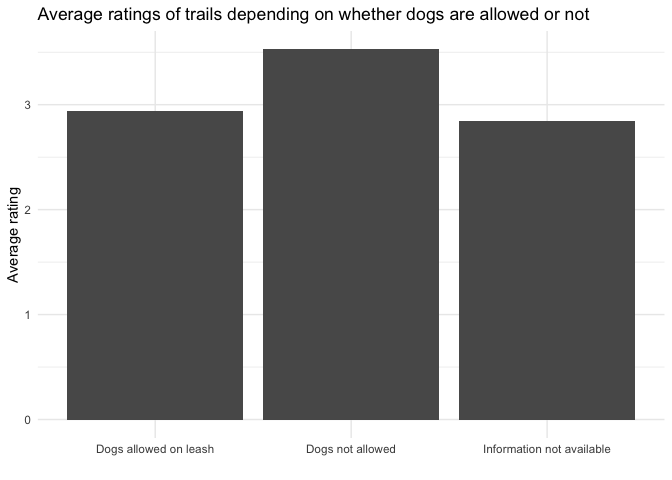# Washington Trails 🥾

Frie 11/24/2020

Thanks to the data, no GIFs this time (unlike last time)! Instead, we have some nice theming, simple but yet effective bar plots and a meme embedded into a ggplot! You can find a list of links that were shared in our Slack channel during the hangout at the end of this post.

Enjoy!

# A doge within a correlation plot

By Long Nguyen

``````library(tidyverse)
library(correlation)
library(tidytuesdayR)
``````
``````##
``````
``````theme_set(
theme_minimal(base_size = 15,
base_family = "FantasqueSansMono Nerd Font") +
theme(panel.ontop = TRUE,
panel.grid = element_line(colour = "#EDEAC2"),
panel.grid.minor = element_blank(),
panel.grid.major.y = element_blank(),
plot.background = element_rect(fill = "#EDEAC2"),
plot.margin = margin(30, 30, 30, 30),
plot.title.position = "plot",
plot.caption.position = "plot")
)

hike_data_cleaned <- hike_data %>%
rownames_to_column("id") %>%
mutate(location_general = str_replace_all(location, "(.*)\\s[-][-].*", "\\1"),
length_total = parse_number(length) * (str_detect(length, "one-way") + 1),
gain = as.integer(gain),
highpoint = as.numeric(highpoint),
rating = as.numeric(rating))
hike_data_long <- hike_data_cleaned %>%
unnest(features, keep_empty = TRUE)
hike_data_onehot <- hike_data_long %>%
mutate(n = 1L) %>%
pivot_wider(names_from = features, values_from = n) %>%
select(-`NA`) %>%
mutate(across(everything(), ~ replace_na(.x, 0L)))
hike_data_onehot %>%
select(rating, `Dogs allowed on leash`:Summits) %>%
correlation::correlation() %>%
filter(Parameter1 == "rating") %>%
ggplot(aes(r, fct_reorder(Parameter2, r))) +
geom_col(aes(fill = r > 0), width = .5, show.legend = FALSE) +
scale_x_continuous(position = "top") +
scale_fill_manual(values = c("#A36B2B", "#2686A0")) +
as.raster(),
.102, .143, 1.5, 6, interpolate = TRUE) +
labs(x = NULL, y = NULL,
title = "Correlation of User Ratings with Features",
subtitle = "of Washington Hiking Trails",
caption = "Data: Washington Trails Association")
``````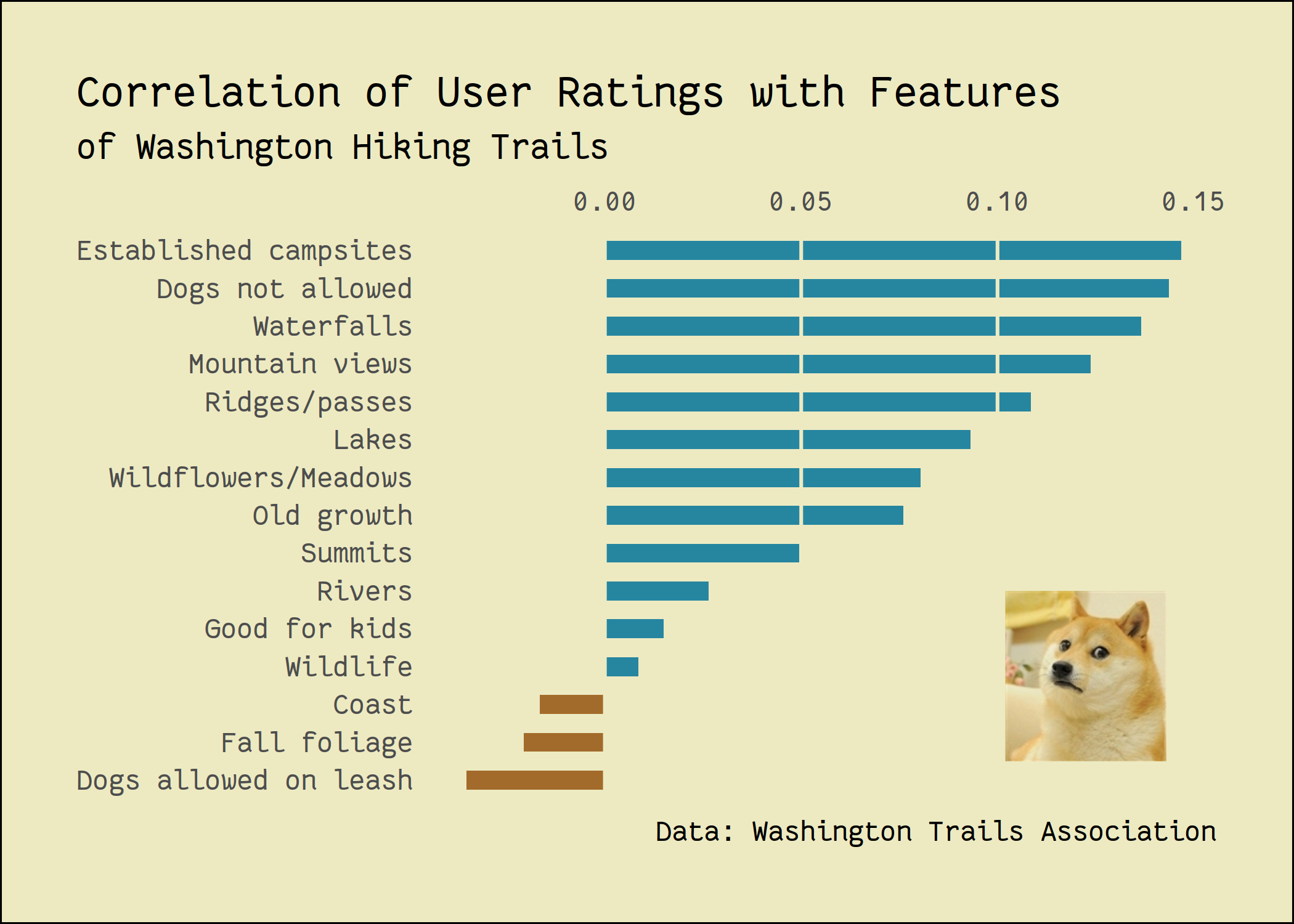My low-effort contribution at this week's @CorrelAid #TidyTuesday meetup.
Alternative title: “Washington hikers HATE dogs” (some highly circulating tabloid, probably)#rstats #dataviz pic.twitter.com/7Y0AkB9PZh

— Long Nguyen (@long39ng) November 25, 2020

# Frequency of trail features

By Sylvi Rzepka

``````library(ggplot2)
library(hrbrthemes)
library(tidyverse)
library(ggtext)
``````
``````hike_data <- readr::read_rds(url('https://raw.githubusercontent.com/rfordatascience/tidytuesday/master/data/2020/2020-11-24/hike_data.rds'))

hike_data_clean<- hike_data %>%
tidyr::unnest(features)

plot4<-ggplot(hike_data_clean, aes(x=fct_rev(fct_infreq(features)))) + # ordered ascendingly
geom_bar(stat="count", fill="bisque3", color="bisque4") +
# Highlighting just a couple of features
scale_x_discrete(labels=c("Coast", "", "", "", "", "", "Ridges/Passes", "", "","", "","","", "", "Mountain \n Views")) +
# edit the theme
theme(text = element_text(family = "Andale Mono"), legend.position = "none", # change all text font and move the legend to the bottom
panel.grid = element_line(color="white"),  # change the grid color and remove minor y axis lines
plot.caption = element_text(hjust = 0.5, size = 8, color = "bisque4"), # remove x-axis text and edit the caption (centered and brown)
panel.grid.major = element_blank(),
panel.background = element_rect(fill="lightblue"),
plot.title = element_text(size = 24),
plot.subtitle = element_markdown(size=8, family = "Helvetica", color = "bisque4")) + # make the title bigger and edit the subtitle (font)
# title
labs(title = "On a mountain high",
subtitle = "Mountain Views, dogs on a leash allowed, and Wildflowers top 3 features of Washington Trails.",
x = "Most frequent features of hikes", y=NULL,
caption = "DataViz by @SylviRz for #TidyTuesday, Data: Washington Trails Association")
plot4
``````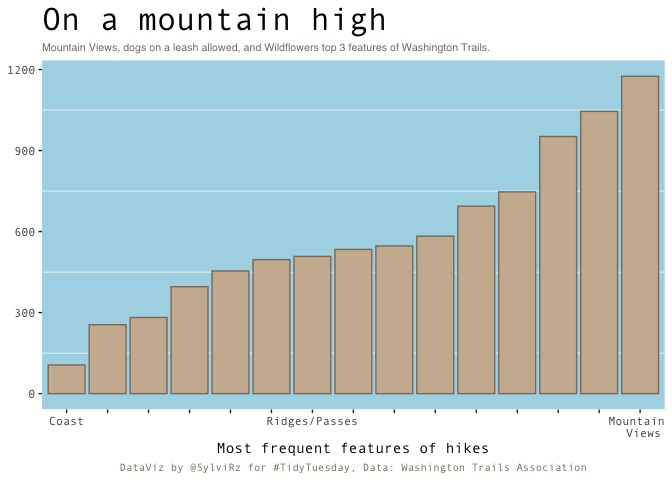By Fodil

# Trail ratings at different locations

By Andreas Neumann

``````library(ggplot2)
library(tidyverse)
library(plyr)
library(ggrepel) #for arrows and labels
``````
``````hike_data <- readr::read_rds(url('https://raw.githubusercontent.com/rfordatascience/tidytuesday/master/data/2020/2020-11-24/hike_data.rds'))

##Data cleaning as proposed##
clean_hike_data <- hike_data %>%
mutate(
trip = case_when(
grepl("roundtrip",length) ~ "roundtrip",
grepl("one-way",length) ~ "one-way",
grepl("of trails",length) ~ "trails"),

length_total = as.numeric(gsub("(\\d+[.]\\d+).*","\\1", length)) * ((trip == "one-way") + 1),

gain = as.numeric(gain),
rating= as.numeric(rating),
highpoint = as.numeric(highpoint),

location_general = gsub("(.*)\\s[-][-].*","\\1",location))

##Extra table to calculate mean rating for each region##
check2<-ddply(clean_hike_data, .(location_general), summarize,  rating=mean(rating))

##Table 1: no mean ratings added in plot##
ggplot()+
geom_point(data=clean_hike_data,aes(location_general,rating,size=length_total,col=trip),alpha=0.25)+
labs(y="Ratings", x="location",title = "How popular are Washington´s hiking trails?",
color = "Trail Category:", size="Length in:\nmiles:")+
geom_hline(yintercept = 2.50,color="white") +
geom_vline(xintercept = 0)+
theme(axis.title.y = element_text(color = "white"),
axis.title.x = element_text(color = "white", margin = margin(10, 0, 0, 0)),
axis.text.y = element_text(color = "#CCCCCC", size = 12),
axis.ticks.y = element_blank(),
axis.text.x = element_text(hjust = 0, color = "white"),
panel.grid.major = element_line(linetype = "blank"),
panel.grid.minor = element_blank(),
panel.background = element_rect(fill = "#333333", color = NA),
plot.background = element_rect(fill = "#333333", color = NA),
plot.title = element_text(hjust = 0.5),
legend.background = element_rect(fill = "#333333", color = NA),
legend.text = element_text(color = "white"),
legend.title = element_text(color = "white"),
legend.key = element_rect(fill = "#333333"),
title = element_text(colour = "#FFFFFF"))+coord_flip()
``````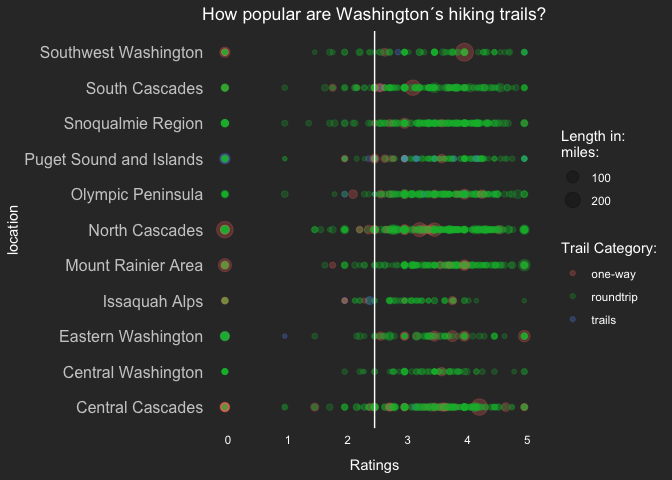``````##Table 2: mean ratings added##
ggplot()+
geom_point(data=clean_hike_data,aes(location_general,rating,size=length_total,col=trip),alpha=0.25)+
labs(y="Ratings", x="location",title = "How popular are Washington´s hiking trails?",
color = "Trail Category:", size="Length in:\nmiles:")+
geom_hline(yintercept = 2.50,color="white") +
geom_point(data=check2, aes(location_general, rating,size=50),shape=17,alpha=1500,color="darkgrey")+
ggrepel::geom_text_repel(data=check2, aes(location_general, rating),segment.color = "#CCCCCC", colour = "grey",label = "average rating")+
guides(size = guide_legend(override.aes = list(shape = 1)))+
geom_vline(xintercept = 0)+
theme(axis.title.y = element_text(color = "white"),
axis.title.x = element_text(color = "white", margin = margin(10, 0, 0, 0)),
axis.text.y = element_text(color = "#CCCCCC", size = 12),
axis.ticks.y = element_blank(),
axis.text.x = element_text(hjust = 0, color = "white"),
panel.grid.major = element_line(linetype = "blank"),
panel.grid.minor = element_blank(),
panel.background = element_rect(fill = "#333333", color = NA),
plot.background = element_rect(fill = "#333333", color = NA),
plot.title = element_text(hjust = 0.5),
legend.background = element_rect(fill = "#333333", color = NA),
legend.text = element_text(color = "white"),
legend.title = element_text(color = "white"),
legend.key = element_rect(fill = "#333333"),
title = element_text(colour = "#FFFFFF"))+coord_flip()
``````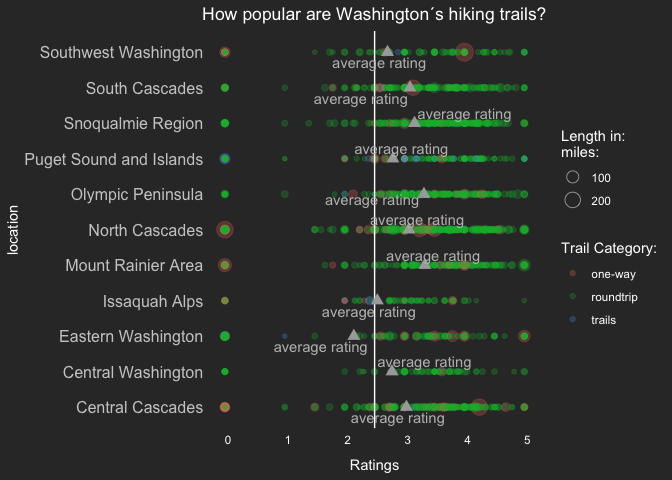# Trail ratings, highest elevation and trail type

By Sarah Wenzel

``````library(tidyverse)
# Data cleaning from Github
clean_hike_data <- hike_data %>%
mutate(
trip = case_when(
grepl("roundtrip",length) ~ "roundtrip",
grepl("one-way",length) ~ "one-way",
grepl("of trails",length) ~ "trails"),
length_total = as.numeric(gsub("(\\d+[.]\\d+).*","\\1", length)) * ((trip == "one-way") + 1),
gain = as.numeric(gain),
highpoint = as.numeric(highpoint),
rating = as.numeric(rating),
location_general = gsub("(.*)\\s[-][-].*","\\1",location)
)
ggplot(aes(x=rating,y=highpoint,color=trip), data=clean_hike_data) +
geom_point() +
theme_classic() +
scale_color_manual(name = "Trail Type", values = c("one-way" = "greenyellow", "roundtrip" = "chartreuse4", "trails"="orange4"), labels = c("One-Way", "Roundtrip", "Trails")) +
scale_y_continuous(name="Highest Point", breaks=seq(0,12000,2000), limits=c(0, 12276)) +
scale_x_continuous(name="Trail Rating", breaks=seq(0,5,1))
``````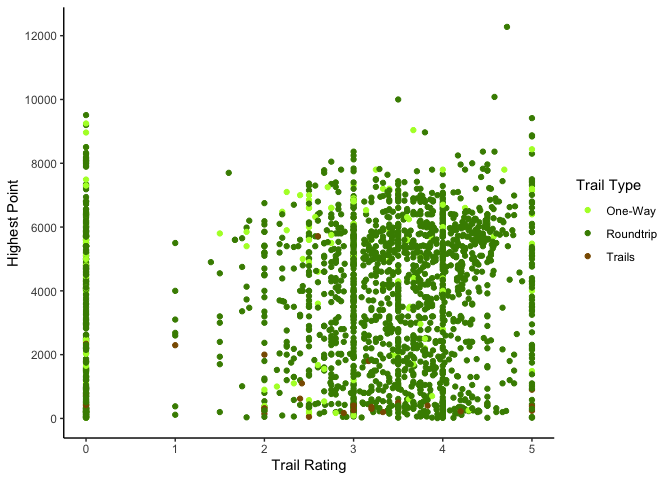# Average rating for dogs-allowed and dogs-not-allowed trails

By Frie Preu

``````library(ggplot2)
library(tidyverse)
``````
``````hike_data\$id <- 1:nrow(hike_data)

# unnest features
hike <- hike_data %>%
tidyr::unnest(features, keep_empty = TRUE)

# dogs allowed / not allowed
hike_dogs <- hike %>%
mutate(dogs_info = stringr::str_extract(features, "Dogs (not )?allowed(.+)?")) %>%
filter(!is.na(dogs_info))

# make sure there are no cases where both dogs are allowed and not
hike_dogs %>% dplyr::count(id) %>% arrange(desc(n))# for sure there is one weird case!!
``````
``````## # A tibble: 1,299 x 2
##       id     n
##    <int> <int>
##  1  1618     2
##  2     1     1
##  3     2     1
##  4     3     1
##  5     4     1
##  6     5     1
##  7     6     1
##  8     7     1
##  9     8     1
## 10     9     1
## # … with 1,289 more rows
``````
``````hike_dogs <- hike_dogs %>% filter(id != 1618) # drop it

hike_data <- hike_data %>%
left_join(hike_dogs %>% select(id, dogs_info), by = "id") %>%
replace_na(list(dogs_info = "Information not available")) %>%
ungroup()

hike_data %>%
dplyr::group_by(dogs_info) %>%
dplyr::summarize(avg_rating = mean(as.numeric(rating), na.rm = TRUE)) %>%
ggplot(aes(x = dogs_info, y = avg_rating))+
geom_col()+
theme_minimal()+
labs(y = "Average rating", x = "", title = "Average ratings of trails depending on whether dogs are allowed or not")
``````
``````## `summarise()` ungrouping output (override with `.groups` argument)
``````